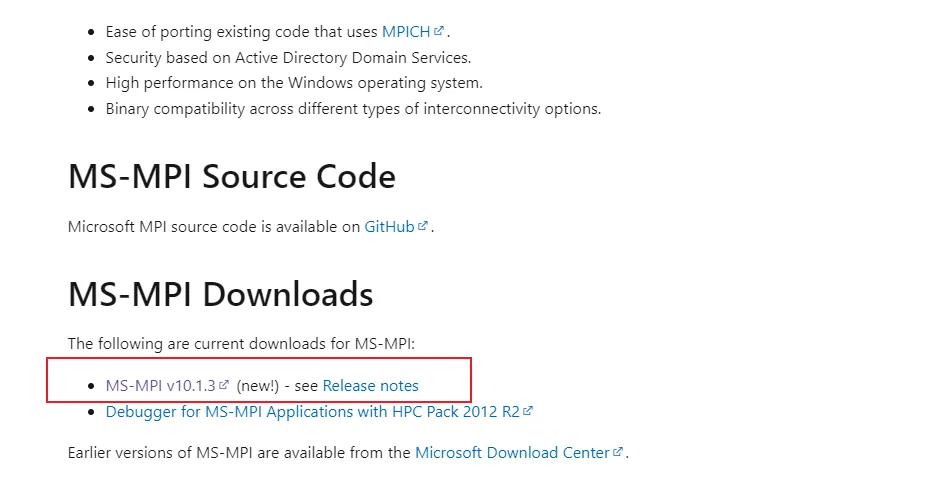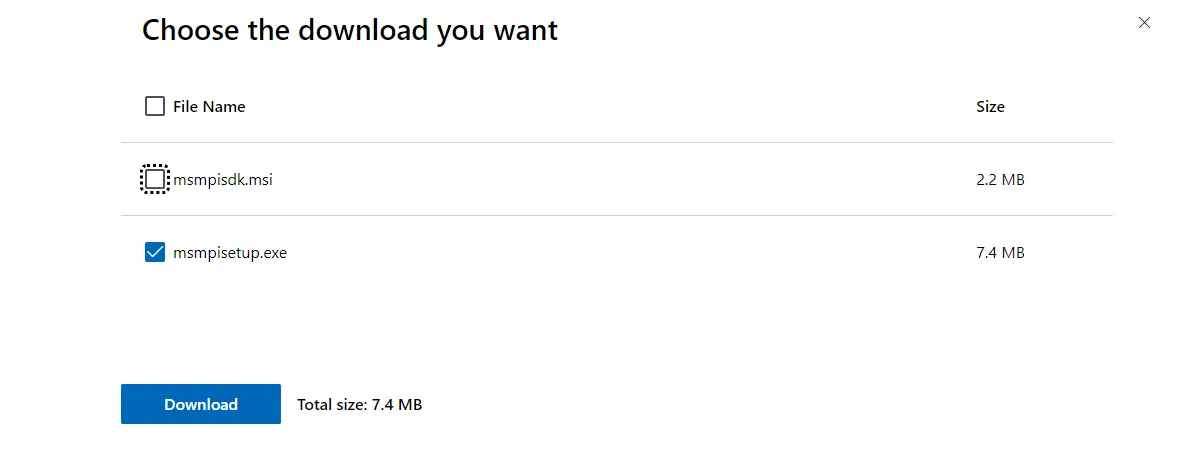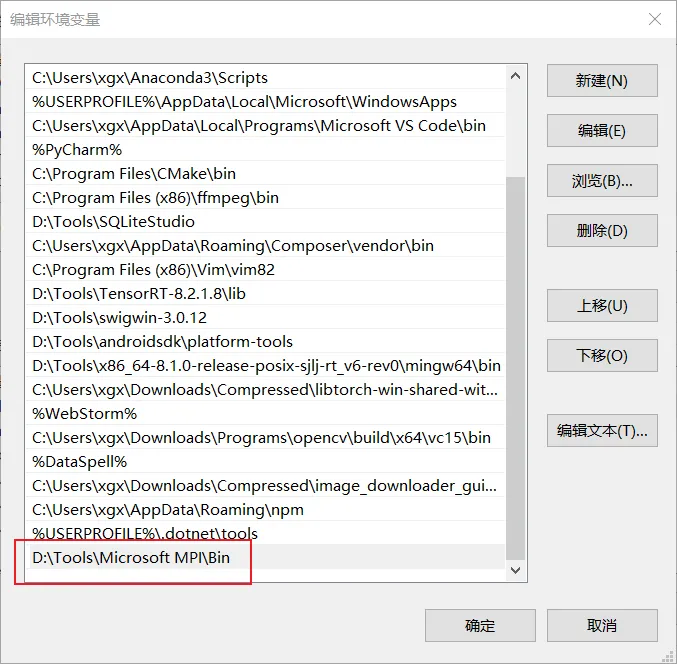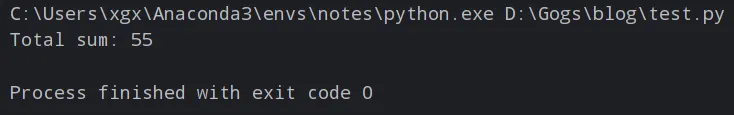欢迎访问我的网站，希望内容对您有用，感兴趣的可以加入免费知识星球。

# Python的一个并行计算库

2个月前 (10-09) 284次浏览

## 安装MPI

https://learn.microsoft.com/en-us/message-passing-interface/microsoft-mpi## 安装mpi4py

``pip install mpi4py``

## 基本使用

``````from mpi4py import MPI

# 初始化MPI环境
comm = MPI.COMM_WORLD

# 获取当前进程的排名和总进程数
rank = comm.Get_rank()
size = comm.Get_size()

# 定义数组
data = [1, 2, 3, 4, 5, 6, 7, 8, 9, 10]

# 计算每个进程的部分和
part_sum = sum(data[rank::size])

# 将部分和发送给主进程
sums = comm.gather(part_sum, root=0)

# 主进程将所有部分和相加得到最终结果
if rank == 0:
total_sum = sum(sums)
print("Total sum:", total_sum)``````